Courses

# Test: Properties Of Determinants

## 10 Questions MCQ Test Mathematics (Maths) Class 12 | Test: Properties Of Determinants

Description
This mock test of Test: Properties Of Determinants for JEE helps you for every JEE entrance exam. This contains 10 Multiple Choice Questions for JEE Test: Properties Of Determinants (mcq) to study with solutions a complete question bank. The solved questions answers in this Test: Properties Of Determinants quiz give you a good mix of easy questions and tough questions. JEE students definitely take this Test: Properties Of Determinants exercise for a better result in the exam. You can find other Test: Properties Of Determinants extra questions, long questions & short questions for JEE on EduRev as well by searching above.
QUESTION: 1

### If the value of the determinant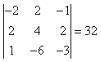, then the value of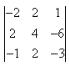is​

Solution:

Determinant = -2(-12 + 12) -2(-6 - 2) +1(-12 - 4)
= - 2(0) - 2(-8) + 1(16)
= 0 + 16 + 16
= 32

QUESTION: 2

### The value of x, if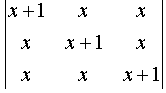= 0 , is​

Solution: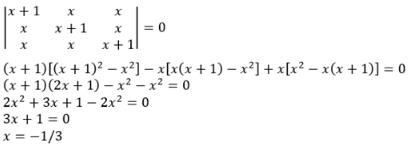QUESTION: 3

### If the value of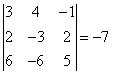, then the value of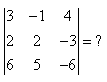Solution:

If you interchange (switch) two rows (or columns) of a matrix A to get B, then det(B) = –det(A). Hence, the determinant is -(-7)

= 7

QUESTION: 4

The operation that will simplify the Determinant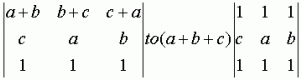by taking (a+b+c) common from the first Row is​

Solution:

R1 ------> R1 + R2, taking (a+b+c) common from the first row, we get the resultant matrix.

QUESTION: 5

The value of the determinant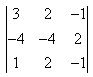is

Solution:

|A|=3((4)-(4))-2((-4)-(2))-1((-8)-(-4))
|A|= -2(-6)-(-6)
|A|= -2(0)
=0

QUESTION: 6

The value of the Δ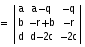Solution:
QUESTION: 7

If the value of the determinant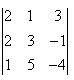is equal to 14, then the value of the determinant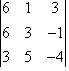is equal to​

Solution:

Taking 3 common from the column I in second determinant, we get the 14 * 3 = 42

QUESTION: 8

The value of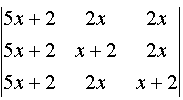is

Solution:

A = {(5x+2, 2x, 2x) (5x+2, x+2, 2x) (5x+2, 2x, x+2)}
= (5x+2) {(1, 2x, 2x) (1, x+2, 2x) (1, 2x, x+2)}
= (5x+2) [(1(x+2)^2 - 4x^2) - 2x(x+2 - 2x) + 2x(2x - x - 2)]
= (5x+2) [(x+2 - 2x)(x+2+2x)-2x(2 - x) + 2x(x-2)]
= (5x+2) [(2-x)(3x+2) -2x(2-x) -2x(2-x)]
= (5x+2) (2-x)[3x+2-2x-2x]
= (5x+2) (2-x)2

QUESTION: 9

Given ​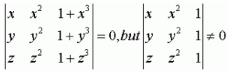Solution: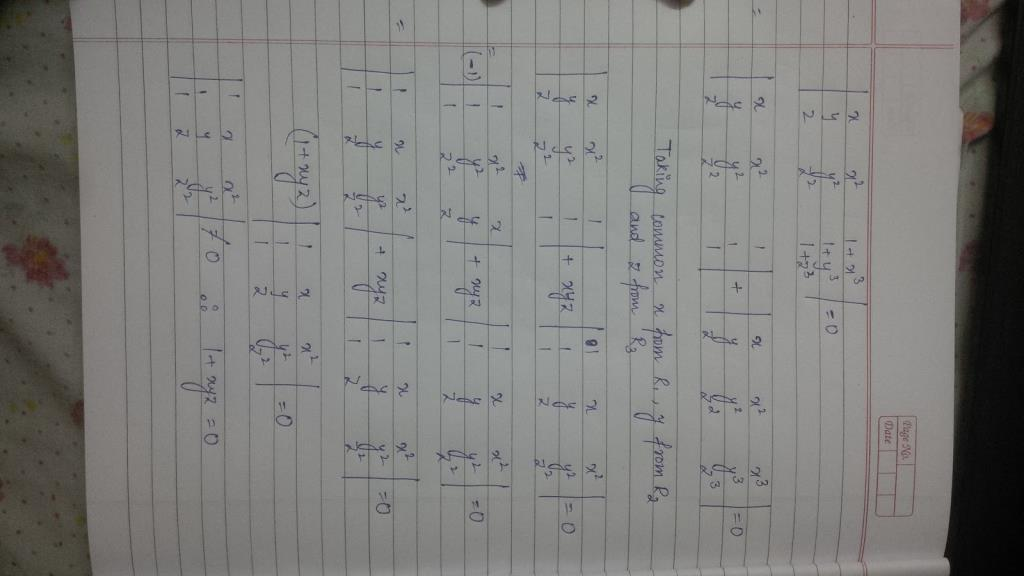QUESTION: 10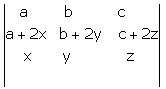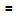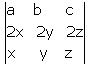+ Δ. then Δ is

Solution: